The problem posits the simple linear regression model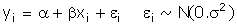The simple correlation coefficient is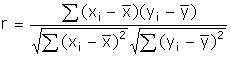1. Notice that there is no presumption about causation between x and y.  This is quite different from the coefficient of determination in a regression model.  In a regression model y is always understood to be the dependent variable.  The coefficient of determination will depend on the choice of left hand side variable.  However, if y is taken to be the dependent variable, then the square of the correlation coefficient and the coefficient of determination will be equal in a simple linear regression model.  If you wanted to use this fact in your answers then you need to prove the equality first.

2. When told to prove or demonstrate something you cannot use the result to be proven in the proof!

3. When told to prove or demonstrate something you cannot pull results out of thin air without showing where the result came from or giving attribution.

The Likelihood Ratio Test for Linear Restrictions on the Regression Coefficients

For the maintained model we have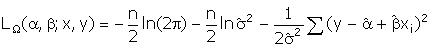The maximum likelihood estimator of the error variance under the null hypothesis is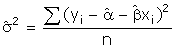Making the substitution, the log likelihood under the null reduces to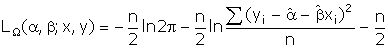For the restricted model, ω, we have by analogy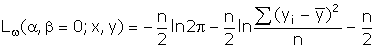The likelihood ratio statistic is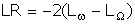Making the substitutions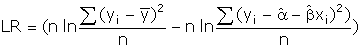The maximum likelihood estimator of the intercept is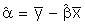Making this substitution and doing some rearranging gives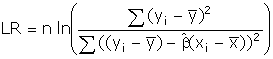In the next steps you should expand the square in the denominator, then multiply the numerator and denominator by the inverse of the sum of squared deviations of y about its mean.  If you do the algebra correctly then you will obtain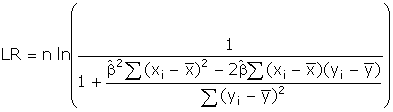The maximum likelihood estimator for the slope coefficient is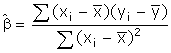Substitute this expression into the LR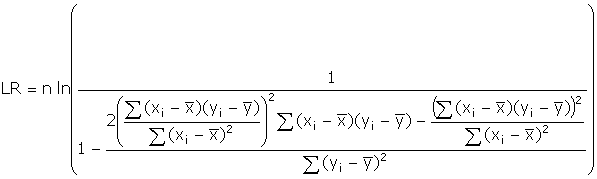With some rearranging and applying the definition of the correlation coefficient you will get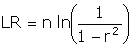Which was the desired result.

The Wald Test for Linear Restrictions

We begin by making use of the definition of the Wald test.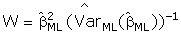The estimate of the variance of the ML estimator for the slope is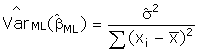Utilizing some of the steps from the answer to the LR question (substituting in for the estimators for the intercept and slope) we can rewrite the estimate of the error variance in the following way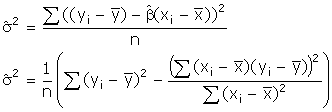Making the substitution into the Wald statistic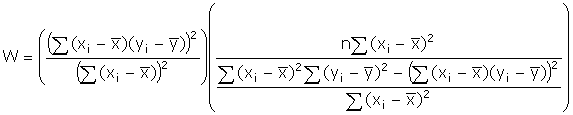With some cancellations and rearranging you will obtain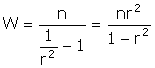Which was the desired result.

The Lagrange Multiplier Test for Linear Restrictions

Recall the likelihood function from the section on the likelihood ratio test.  Differentiate that function with respect to each of the unknown parameters.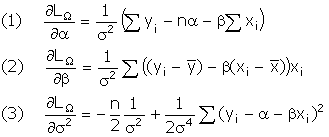(a)  We will also need the inverse of the negative of the information matrix.  Recall that the information matrix is the Hessian for the likelihood function.  Since (1) and (3) are both zero when evaluated at the restrictions,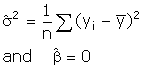,

we only need one term in the information matrix.  Namely, after first substituting the ML estimator for the intercept into (2),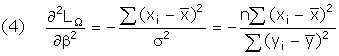The second equality in (4) comes from evaluating the derivative at the restrictions.

(b) From the observation in (a) and the definition of the LM test in the text we can write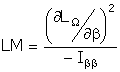From (2) we can write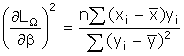Plugging into the test statistic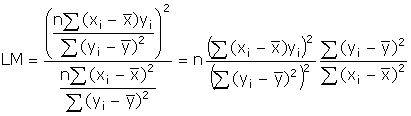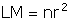Which is the desired result.